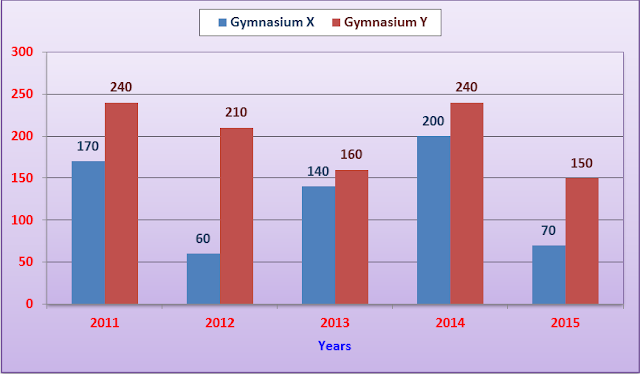# High Level Data Interpretation Quiz for SBI PO Mains

High Level Data Interpretation Quiz for SBI PO Mains
Directions (1 – 5) : Study the following table carefully to answer the questions given below it :
Percentage of students passed with respect to those appeared from six states over the years in an admission test.
 State Year 2011 2012 2013 2014 2015 2016 Bihar 40 37 27 38 26 33 UP 37 29 27 39 26 33 MP 32 45 28 36 40 24 AP 29 37 42 45 30 36 Punjab 35 26 38 42 34 29 Jharkhand 39 43 36 29 35 41
1. If the number of students appeared from UP in 2015 was 15000 and was more than that in 2014 by 25%, then what was the difference between the number of qualified students in UP in 2014 and that in 2015 respectively ?
A) 700
B) 650
C) 780
D) 680
E) 800
2. In the year 2011, if 30% of passed out students who got above 60% marks was 1740 from AP, then how many students could not pass from AP ?
A) 14500
B) 14200
C) 15000
D) 18000
E) None of these
3. If the number of qualified students from MP in 2012 and 2014 were in the ratio of 2 : 3, then w hat was the ratio of students who appeared in these years ?
A) 8 : 15
B) 8 : 25
C) 7 : 15
D) 15 : 16
E) None of these
4. If the number of students appeared from MP in 2012 and that from Panjab in 2014 was 18000 and 21000 respectively, then what is the difference between the number of passed out students of those states in those years ?
A) 680
B) 750
C) 720
D) 800
E) 850
5. If the number of appeared students from Bihar in 2011 was 28780 and 15% of the total number of appeared students get above 45% marks and below 60% and 25% of qualified students get above 60% marks, then the number of students who get above 60% marks is approximately what per cent of the total number of students who get above 45% marks and below 60% marks ?
A) 63%
B) 61%
C) 59%
D) 67%
E) 57%
Directions (6 – 10): Study the following bar chart and answer the questions
The bar-graph shows the total number of members enrolled in different years from 2011 to 2015 in two gymnasiums x and y. Based on this bar-graph, solve the following questions.6. The number of members enrolled in gymnasium x from 2012 to 2015 together is what per cent more than the number of members enrolled in gymnasium y in 2014 and 2015 together (rounded off to two digit decimal places)
A) 20.51%
B) 18.51%
C) 15.51%
D) 22.51%
E) None of these
7. The number of members of gymnasium x in 2012 is what percent of the number of members of gymnasium y in 2015 ?
A) 50%
B) 45%
C) 40%
D) 55%
E) 65%
8. If in the year 2016, there is 30% increase in the total number of members enrolled in 2015 in both gymnasiums then find the total number of members enrolled in 2016 ?
A) 286
B) 292
C) 275
D) 272
E) 278
9. The total number of members enrolled in gymnasium y in 2014 and 2015 together is what per cent more than the number of members enrolled in gymnasium x in 2011 and 2015 together ?
A) 65%
B) 62.5%
C) 72%
D) 64%
E) None of these
10. The ratio of the total number of members of both gymnasiums in 2012 to the total number of members in 2015 of both gymnasiums is :
A) 22 : 27
B) 21 : 11
C) 27 : 22
D) 11 : 21
E) 13 : 25
Solutions:
1. C) The number of appearedstudents from UP in 2015 = 15000
The no. of appeared students from UP in 2014 = 15000 x 100/125 = 12000
Therefore, required difference = 12000 x 39/100 – 150000 x 26/100 = 4680 – 3900 = 780
2. B) 30% of passed out students from AP who got above 60% marks = 1740
Therefore total passed out students from AP = 1740 x 100/30 = 5800
Therefore no. of students who could not pass = 5800  71/29 = 14200
3. A) Required ratio = 2 x (100/45) : 3 x (100/36) = 8 : 15
4. C) Required difference = 21000 x 42/100 – 18000 x 45/100 = 8820 – 8100 = 720
5. D) Required percentage = [28780 x (40/100) x (25/100)]/[28780 x (15/100)] x 100 = 200/3 = 66.66% ≈ 67%
6. A) Total no. of members enrolled in Gymnasium X from 2012 to 2015 = 60 + 140 + 200 + 70 = 470
Total no. of members enrolled in Gymnasium Y from 2014 to 2015 = 240 + 150 = 390
Difference = 470 – 390 = 80
Therefore required percentage = 80/390 x 100 = 20.51% more
7. C) Required % = 60/150 x 100 = 40%
8. A) Total no. of members enrolled in 2016 = 130% of (150 + 70) = 130/100 x 220 = 286
9. B) Total no. of members enrolled in Gym Y in 2014 and 2015 = 240 + 150 = 390
Total no. of members enrolled in Gym x in 2011 and 2015 = 170 + 70 = 240
Therefore difference = 390 – 240 = 150
Therefore required % = 150/240 x 100 = 62.5%
10. C) Required ratio = (60 + 210)/(70 + 150) = 270/220 = 27 : 22